CDA数据科学研究院 CDA考试中心 CDA人工智能学院 企业服务 关于CDA

#全国校区## 分析20万条弹幕告诉你，8.9分的高分剧《隐秘的角落》到底好看在哪儿？2020-06-3001拿拍电影的态度拍网剧 ,不好看才怪

该剧改编自紫金陈推理小说《坏小孩》 ，讲述了沿海小城的三个孩子在景区游玩时无意拍摄记录了一次谋杀，他们的冒险也由此展开。扑朔迷离的案情，将几个家庭裹挟其中，带向不可预知的未来......剧情不拖沓，演技全员在线

而整部剧中，无论是从挑大梁的秦昊，到三位小演员，还是王景春、张颂文等一众演员都奉献出了无可挑剔的演技。

令人印象深刻的配乐02豆瓣8.9分 年度国剧之光！

首先，我们看到豆瓣的数据。这部剧一开播在豆瓣评分就冲上9.0分，一度冲到9.2分，随着剧集完结，目前稳定在8.9分，已经有45万余人进行了评分。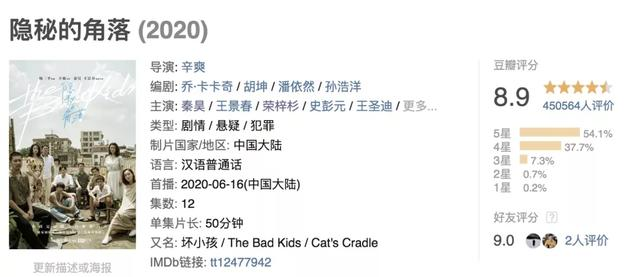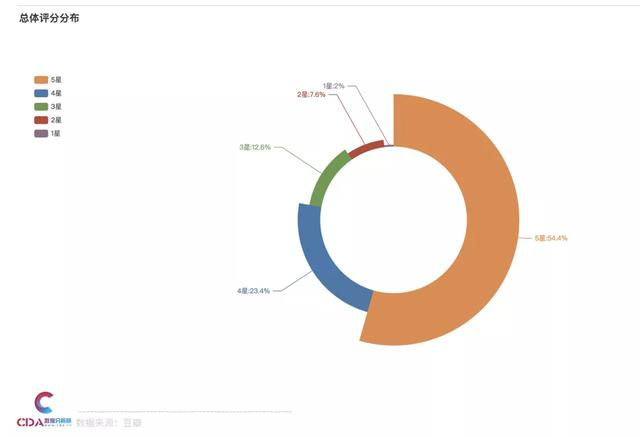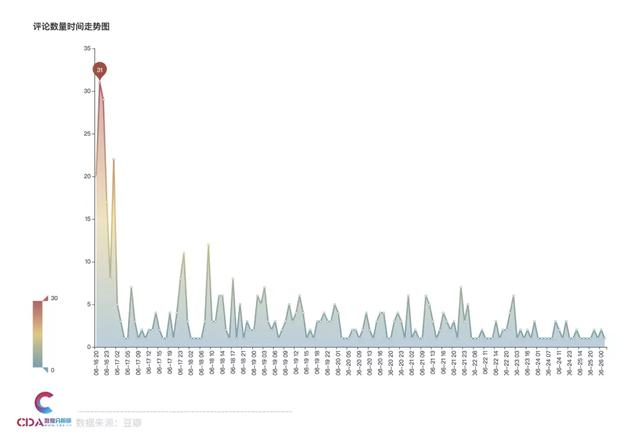评论中提及主演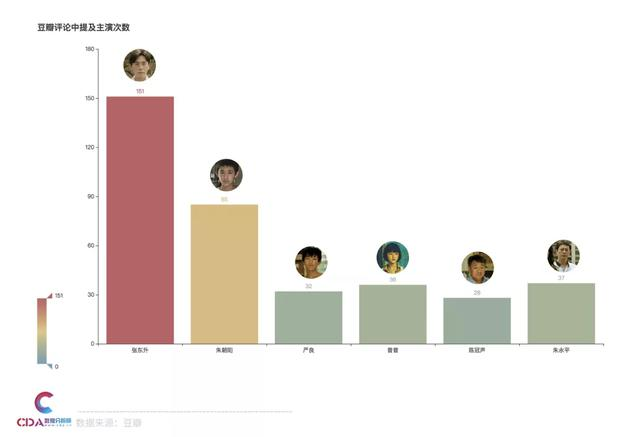主演评价分布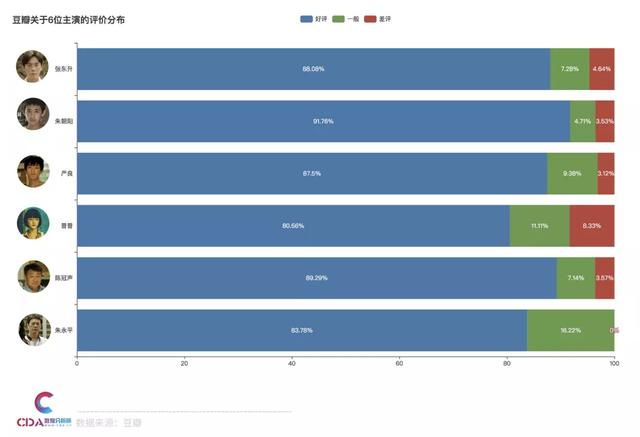0320万条弹幕告诉你

接下来我们分析一下《隐秘的角落》在爱奇艺的弹幕数据，我们分析整理了全部12集的弹幕，共200672条。下面看到分析结论：

用户使用的弹幕角色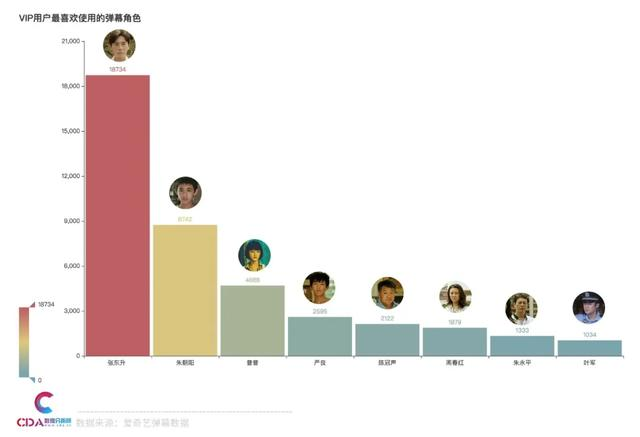可以看到，这方面张东升在这方面是榜首。其次是朱朝阳，然后可爱的小妹妹普普位居第三。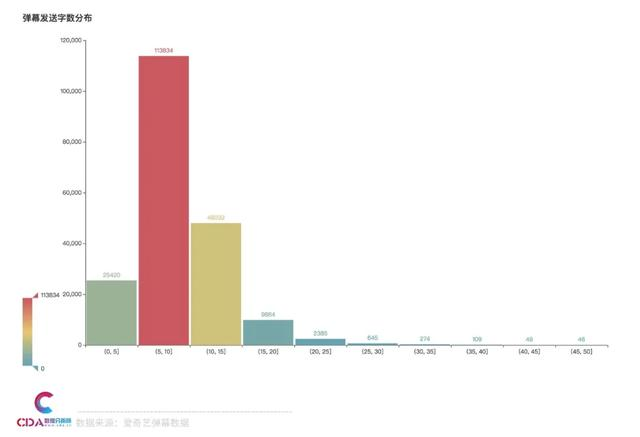整体弹幕词云接下来，我们分别看到几位主演的人物弹幕词云吧。

张东升朱朝阳普普严良04教你用Python分析爱奇艺弹幕数据

我们使用Python获取并分析爱奇艺的弹幕数据，整个数据分析的流程分为以下三个部分：

```# 导入库
import pandas as pd
import os
import jieba

from pyecharts.charts import Bar, Pie, Line, WordCloud, Page
from pyecharts import options as opts
from pyecharts.globals import SymbolType, WarningType
WarningType.ShowWarning = False

import stylecloud
from IPython.display import Image```

```# 文件列表
data_list = os.listdir('../data/')
data_list```

```['df_第一集.csv',
'df_第七集.csv',
'df_第三集.csv',
'df_第九集.csv',
'df_第二集.csv',
'df_第五集.csv',
'df_第八集.csv',
'df_第六集.csv',
'df_第十一集.csv',
'df_第十二集.csv',
'df_第十集.csv',
'df_第四集.csv']```

```# 存储数据
df_all = pd.DataFrame()

# 循环写入
for i in data_list:
df_one = pd.read_csv(f'../data/{i}', engine='python', encoding='utf-8', index_col=0)
df_all = df_all.append(df_one, ignore_index=False)

# 打印数据集大小
print(df_all.shape) ```

`(200672, 6)`

```# 预览数据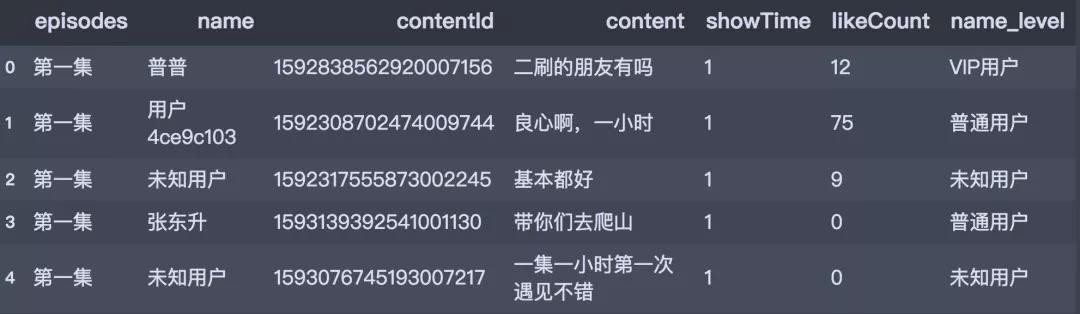```# 字符串处理
df_all['name'] = df_all.name.str.strip()

def transform_name(x):
if x=='张东升' or x=='朱朝阳' or x=='普普' or x=='严良' or x=='陈冠声' or x=='周春红' or x=='朱永平' or x=='叶军':
return 'VIP用户'
elif x=='未知用户':
return '未知用户'
else:
return '普通用户'

# 新增列
df_all['name_level'] = df_all.name.apply(transform_name)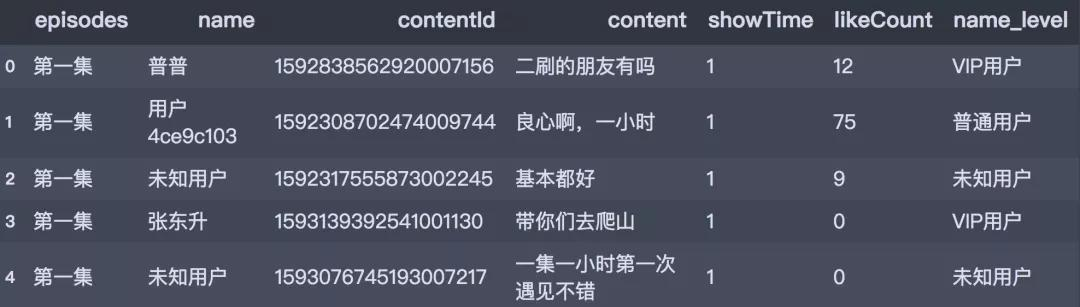1. 用户最喜欢使用的弹幕角色-条形图
2. 弹幕发送字数分布-条形图
3. 弹幕角色-词云图

```level_num = df_all.name_level.value_counts()
level_num ```

```未知用户     157722
VIP用户     41127

Name: name_level, dtype: int64```

```data_pair =  [list(z) for z in zip(level_num.index.tolist(), level_num.values.tolist())]

# 绘制饼图
pie1 = Pie(init_opts=opts.InitOpts(width='1350px', height='750px'))
pie1.set_global_opts(title_opts=opts.TitleOpts(title='弹幕发送人群等级分布'),
legend_opts=opts.LegendOpts(orient='vertical', pos_top='15%', pos_left='2%'))
pie1.set_series_opts(label_opts=opts.LabelOpts(formatter="{b}:{d}%"))
pie1.set_colors(['#3B7BA9', '#6FB27C', '#FFAF34'])
pie1.render() ```

name字段中标注了用户发送弹幕时候使用的弹幕角色，统计并筛选不同弹幕角色的使用频次。

```role_num = df_all.name.value_counts()[1:9]
role_num```

```

Name: name, dtype: int64

```

```# 柱形图
bar1 = Bar(init_opts=opts.InitOpts(width='1350px', height='750px'))
bar1.set_global_opts(title_opts=opts.TitleOpts(title="VIP用户最喜欢使用的弹幕角色"),
visualmap_opts=opts.VisualMapOpts(max_=18734),
)
bar1.render() ```

content字段记录了用户评论的弹幕信息，此处根据这个字段计算字数并按照步长5进行分箱处理，得到不同字数段下的频次分布。

```word_num = df_all.content.apply(lambda x:len(x))

# 分箱
bins = [0, 5, 10, 15, 20, 25, 30, 35, 40, 45, 50]
word_num_cut = pd.cut(word_num, bins, include_lowest=False).value_counts()
word_num_cut = word_num_cut.sort_index()
word_num_cut```

```(0, 5]       25420
(5, 10]     113834
(10, 15]     48032
(15, 20]      9864
(20, 25]      2385
(25, 30]       645
(30, 35]       274
(35, 40]       109
(40, 45]        49
(45, 50]        46
Name: content, dtype: int64```

```# 柱形图
bar2 = Bar(init_opts=opts.InitOpts(width='1350px', height='750px'))
bar2.set_global_opts(title_opts=opts.TitleOpts(title="弹幕发送字数分布"),
visualmap_opts=opts.VisualMapOpts(max_=113834),
)
bar2.render()```

```
# 定义分词函数
def get_cut_words(content_series):
# 读入停用词表
stop_words = []

with open(r"C:\Users\wzd\Desktop\CDA\CDA_Python\Python文本分析\10.文本摘要\stop_words.txt", 'r', encoding='utf-8') as f:
for line in lines:
stop_words.append(line.strip())

# 添加关键词
my_words = ['秦昊', '张东升', '王景春', '陈冠声', '荣梓杉',
'朱朝阳', '史彭元', '严良', '王圣迪', '普普',
'岳普', '张颂文', '朱永平', '十二集', '十二万',
'十二时辰']

for i in my_words:

# 自定义停用词
my_stop_words = ['真的', '这部', '这是', '一种', '那种',
'哈哈哈']
stop_words.extend(my_stop_words)

# 分词
word_num = jieba.lcut(content_series.str.cat(sep='。'), cut_all=False)

# 条件筛选
word_num_selected = [i for i in word_num if i not in stop_words and len(i)>=2]

return word_num_selected

```

```text1 = get_cut_words(content_series=df_all[df_all.name=='张东升']['content'])
text1[:5] ```

```Building prefix dict from the default dictionary ...
Dumping model to file cache C:\Users\wzd\AppData\Local\Temp\jieba.cache
Prefix dict has been built successfully.
['爬山', '老弟', '十二集', '知足', '伊能静']``````# 绘制词云图
stylecloud.gen_stylecloud(text=' '.join(text1), max_words=1000,
collocations=False,
font_path=r'‪C:\Windows\Fonts\msyh.ttc',
icon_name='fas fa-heart',
size=653,
output_name='./词云图/弹幕角色-张东升词云图.png')
Image(filename='./词云图/弹幕角色-张东升词云图.png')```

OK